Download the eSaral app and start learning from Kota's top IITians and doctors.

# Solid State - JEE Main Previous Year Questions with Solutions

JEE Main Previous Year Papers Questions of Chemistry with Solutions are available at eSaral. Practicing JEE Main chapter wise questions of Chemistry will help the JEE aspirants in realizing the question pattern as well as help in analyzing weak & strong areas.   Simulator Previous Years AIEEE/JEE Mains Questions
Q. The edge length of a face centered cubic cell of an ionic substance is 508 pm. If the radius of the cation is 110 pm, the radius of the anion is :- (1) 144 pm (2) 288 pm (3) 398 pm (4) 618 pm Aieee-10
Ans. (1) radius of cation = 110 pm if cation present 2n OV $a=2\left(r_{+}+r_{-}\right)$ 508 = 2[110 + x] x = 144 pm
Q. Percentages of free space in cubic close packed structure and in body centered packed structure are respectively :- (1) 48% and 26% (2) 30% and 26% (3) 26% and 32% (4) 32% and 48% Aieee-10
Ans. (3) Theory Based
Q. Copper crystallises in fcc lattice with a unit cell edge of 361 pm. The radius of copper atom is:- (1) 181 pm (2) 108 pm (3) 128 pm (4) 157 pm Aieee-11 / JEE-Main-ONLINE 2013
Ans. (3)
Q. In a face centred cubic lattice, atom A occupies the corner positions and atom B occupies the face centre positions. If one atom of B is missing from one of the face centred points, the formula of the compound is :- (1) $\mathrm{A}_{2} \mathrm{B}_{3}$ ( 2) $\mathrm{A}_{2} \mathrm{B}_{5}$ (3) $\mathrm{A}_{2} \mathrm{B}$ (4) $\mathrm{AB}_{2}$ Aieee-11 / JEE-Main-ONLINE 2014
Ans. (2)
Q. Lithium forms body centred cubic structure. The length of the side of its unit cell is 351 pm. Atomic radius of the lithium will be :- (1) 152 pm (2) 75 pm (3) 300 pm (4) 240 pm Aieee-12
Ans. (1)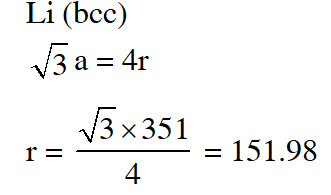Q. The radius of a calcium ion is 94 pm and of the oxide ion is 146 pm. The possible crystal structure of calcium oxide will be :- (1) Octahedral        (2) Tetrahedral          (3) Pyramidal           (4) Trigonal Jee-Main (online)-12
Ans. (1)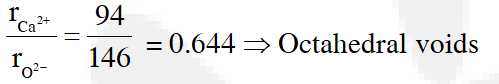Q. Ammonium chloride crystallizes in a body centred cubic lattice with edge length of unit cell of 390 pm. If the size of chloride ion is180 pm, the size of ammonium ion would be: (1) 158 pm (2) 174 pm (3) 142 pm (4) 126 pm Jee-Main (online)-12
Ans. (1)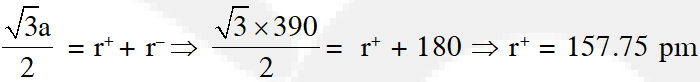Q. A solid has 'bcc' structure. If the distance of nearest approach between two atoms is 1.73 Å, the edge length of the cell is :- (1) 314.20 pm (2) 216 pm (3) 200 pm (4) 1.41 pm Jee-Main (online)-12
Ans. (3)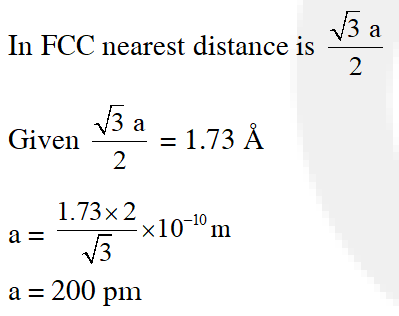Q. Among the following the incorrect statement is :- (1) Density of crystals remains unaffecfted due to Frenkel defect (2) In BCC unit cell the void space is 32% (3) Electrical conductivity of semiconductors and metals increases with increase in temperature (4) Density of crystals decreases due to Schottky defect Jee-Main (online)-12
Ans. (3) Theory Based
Q. In a face centred cubic lattice, atoms of A form the corner points and atoms of B form the face centred points. If two atoms of A are missing from the corner points, the formula of the ionic compound is (1) $\mathrm{AB}_{2}$ ( 2) $\mathrm{AB}_{3}$ (3) $\mathrm{AB}_{4}$ (4) $\mathrm{A}_{2} \mathrm{B}_{5}$ Jee-Main (online)-13
Ans. (3)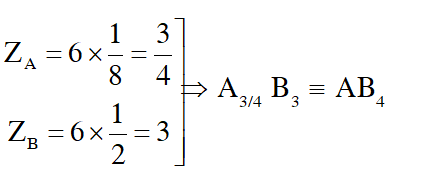Q. Which one of the following statements about packing in solids is incorrect ? (1) Void space in ccp mode of packing is 26% (2) Coordination number in hcp mode of packing is 12 (3) Void space in hcp mode of packing is 32% (4) Coordination number in bcc mode of packing is 8 Jee-Main (online)-13
Ans. (3) Theory based
Q. An element having an atomic radius of 0.14 nm crystallizes in an fcc unit cell. What is the length of a side of the cell ? (1) 0.96 nm (2) 0.4 nm (3) 0.24 nm (4) 0.56 nm Jee-Main (online)-13
Ans. (2)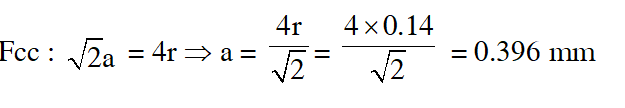Q. Expermentally it was found that a metal oxide has formula $\mathrm{M}_{0.98} \mathrm{O}$. Metal M, is present as $\mathrm{M}^{2+}$ and $\mathbf{M}^{3+}$ in its oxide. Fraction of the metal which exists as $\mathrm{M}^{3+}$ would be :- (1) 7.01%          (2) 4.08%           (3) 6.05%           (4) 5.08 Jee-Main (offline)-13
Ans. (2)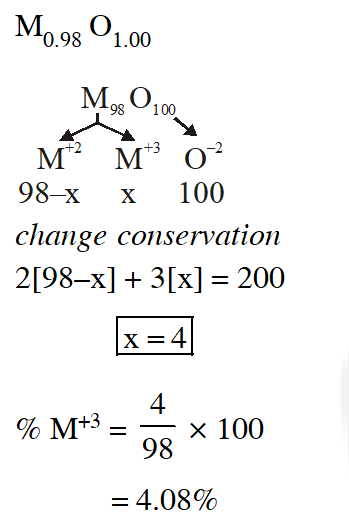Q. The total number of octahedral void(s) per atom present in a cubic close packed structure is :- (1) 1          (2) 2              (3) 3              (4) 4 Jee-Main (online)-14
Ans. (1) Theory based
Q. In a monoclinic unit cell, the relation of sides and angles are respectively (1) $\mathrm{a} \neq \mathrm{b} \neq \mathrm{c}$ and $\alpha \neq \beta \neq \gamma \neq 90^{\circ}$ (2) $a \neq b \neq c$ and $\beta=\gamma=90^{\circ} \neq \alpha$ (3) $a=b \neq c$ and $\alpha=\beta=\gamma=90^{\circ}$ (4) $a \neq b \neq c$ and $\alpha=\beta=\gamma=90^{\circ}$ Jee-Main (online)-14
Ans. (2) Theory based
Q. The appearance of colour in solid alkali metal halides is generally due to : (1) Frenkel defect (2) F-centres (3) Schottky defect (4) Interstitial position Jee-Main (online)-14
Ans. (2) Theory based
Q. CsCl crystallises in body centred cubic lattice. if 'a' is its edge length then which of the following expression is correct : (1) $\mathrm{r}_{\mathrm{Cs}^{+}}+\mathrm{r}_{\mathrm{Cl}^{-}}=\frac{\sqrt{3}}{2} \mathrm{a}$ (2) $\mathrm{r}_{\mathrm{Cs}^{+}}+\mathrm{r}_{\mathrm{Cl}^{-}}=\sqrt{3} \mathrm{a}$ (3) $\quad \mathrm{r}_{\mathrm{Cs}^{+}}+\mathrm{r}_{\mathrm{Cl}^{-}}=3 \mathrm{a}$ (4) $\mathrm{r}_{\mathrm{Cs}^{+}}+\mathrm{r}_{\mathrm{Cl}^{-}}=\frac{3 \mathrm{a}}{2}$ Jee-Main (offline)-14
Ans. (1)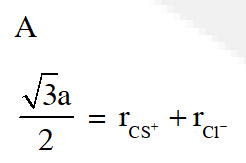Q. A metal crystallises in a face centred cubic structure. If the edge length of its unit cell is 'a', the closest approach between two atoms in metallic crystal will be :- (1) 2a (2) $2 \sqrt{2} \mathrm{a}$ (3) $\sqrt{2} \mathrm{a}$ (4) $\frac{\mathrm{a}}{\sqrt{2}}$ JEE - Main 2017
Ans. (4)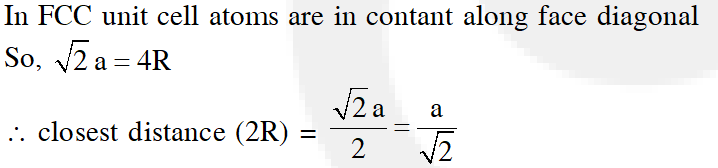Q. Which type of 'defect' has the presence of cations in the interstitial sites ? (1) Vacancy defect (2) Frenkel defect (3) Metal deficiency defect (4) Schottky defect JEE - Main 2018
Ans. (2) In Frenkel defect, some of ion (usually cation due to their small size) missing from their correct position and occupies position in interstitial.

Dec. 7, 2022, 12:07 p.m.
nice Q and increase my confidence
Sakshi
June 7, 2022, 1:51 p.m.
Nice it increase my confidence 😍
Vinam Sharma
April 18, 2022, 11:26 p.m.
its actually increases our confidence which is very very important thanks...
TIGER
April 13, 2022, 8:26 a.m.
plz correct your option of monoclinic...
Feb. 24, 2022, 4:58 p.m.
I am students
Alok
Oct. 25, 2021, 1:09 p.m.
Thankyou so much this helps me a lot
Devisri
Oct. 2, 2021, 7:46 a.m.
Thank you so much it help full to us
SANZU
Sept. 8, 2021, 12:11 p.m.
loved it
Bhakhar Ram
July 17, 2021, 7:25 p.m.
thank you too much this helps me keep it up i'll forward it
Sai
July 17, 2021, 3:22 p.m.
Tqs
Thanks to eSARAL..
June 4, 2021, 9:15 p.m.
Thank you
Aman
March 9, 2021, 4:47 p.m.
My all correct still 80 percentile
Ashwin bhai
Feb. 6, 2021, 7:25 a.m.
🔥🔥🔥
Sai
Jan. 22, 2021, 11:59 a.m.
Aarya
Dec. 30, 2020, 8:44 a.m.
☺️ thank u so much
Anukriti raj vindhyachal
Dec. 24, 2020, 10:57 a.m.
I solved all the question. Thanks esaral
Bamniya
Sept. 24, 2020, 5:28 p.m.
For priti sister
Bamniya
Sept. 24, 2020, 5:26 p.m.
Sister please,but you may be check this question 😎😎😎😎😎😚😚😚😚
yo
Sept. 2, 2020, 2:28 p.m.
GOOD content really
Srigowthami
Aug. 30, 2020, 10:41 a.m.
Xx
Aug. 25, 2020, 4:39 p.m.
Amazing and wonderful questions 😃
suhail dar
Aug. 22, 2020, 3:33 p.m.
really amazing...
Aswin S
July 31, 2020, 10:23 p.m.
Ram
July 18, 2020, 11:13 p.m.
Plzz increase difficulty ..!!
shaikul
July 14, 2020, 8:23 p.m.
Deepna Maria Jimson
July 6, 2020, 4:19 a.m.
Is the 1srt &amp; 2nd questions should be done in that method?? I think that steps are incorrect...
Mahesh
June 21, 2020, 9:25 a.m.
Very good question
Sanvi
June 11, 2020, 6:41 a.m.
Good content
angel
June 9, 2020, 2:55 p.m.
angel
June 9, 2020, 2:41 p.m.
good bits
June 7, 2020, 7:19 a.m.
Good question.
June 7, 2020, 7:18 a.m.
Good question......it really worked for me .........😀😀
Rishi
May 28, 2020, 3:54 p.m.
Sir the answer for question 1is not true We have to use a=2√2r But here u used that a =4r
P Naveen Kumar
May 21, 2020, 7:01 p.m.
Good
Priti
May 11, 2020, 2:02 p.m.
we should have used a= 2√2 r relation in Q 1 ??? as it is mentioned face centered cubic lattice !
SAI CHARAN
April 17, 2020, 6:59 p.m.
sir ans of Q2 is wrong its"D""""""
SAI
April 17, 2020, 6:58 p.m.
sir ans of Q2 is wrong its"D""""""
Pavan
April 8, 2020, 6:18 a.m.
This is very useful to me thank you